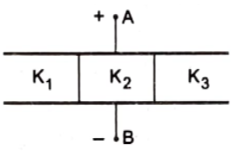Questions

# The space between the plates of a parallel-plate capacitor of capacitance C is filled with three dielectric slabs of identical size as shown in figure. If the dielectric constants of the three slabs are  then find the new capacitanceEasy
A

${\mathrm{C}}_{\mathrm{eq}}=\left({\mathrm{K}}_{1}+{\mathrm{K}}_{2}+{\mathrm{K}}_{3}\right)\frac{\mathrm{C}}{3}$

B

${\mathrm{C}}_{\mathrm{eq}}=\left({\mathrm{K}}_{1}+{\mathrm{K}}_{2}+{\mathrm{K}}_{3}\right)\frac{2\mathrm{C}}{3}$

C

${\mathrm{C}}_{\mathrm{eq}}=\left({\mathrm{K}}_{1}+{\mathrm{K}}_{2}+{\mathrm{K}}_{3}\right)\frac{3\mathrm{C}}{3}$

D

${\mathrm{C}}_{\mathrm{eq}}=\left({\mathrm{K}}_{1}+{\mathrm{K}}_{2}+{\mathrm{K}}_{3}\right)\frac{4\mathrm{C}}{3}$